Courses

# Solving Linear Equations - Matrix Algebra, CSIR-NET Mathematical Sciences Mathematics Notes | EduRev

## Mathematics : Solving Linear Equations - Matrix Algebra, CSIR-NET Mathematical Sciences Mathematics Notes | EduRev

The document Solving Linear Equations - Matrix Algebra, CSIR-NET Mathematical Sciences Mathematics Notes | EduRev is a part of the Mathematics Course Mathematics for IIT JAM, CSIR NET, UGC NET.
All you need of Mathematics at this link: Mathematics

The matrix method of solving systems of linear equations is just the elimination method in disguise. By using matrices, the notation becomes a little easier.

Suppose you have a system of linear equations such as: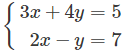The first step is to convert this into a matrix. Make sure all equations are in standard form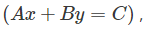and use the coefficients of each equation to form each row of the matrix. It may help you to separate the right column with a dotted line.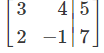Next, we use the matrix row operations to change the 2×2 matrix on the left side to the identity matrix . First, we want to get a zero in Row 1 , Column 2 . So, add 4 times Row 2 to Row 1 .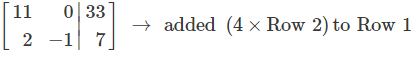Next we want a 1 in the top left corner.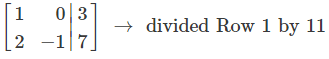Now we want a zero in the bottom left corner.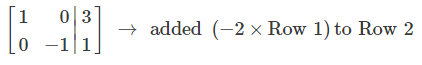Finally, we want a 1 in Row 2 , Column 2 .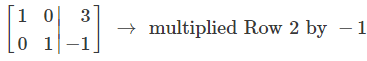Now that we have the 2×2 identity matrix on the left, we can read off the solutions from the right column:

x = 3

y = -1

The same method can be used for nn linear equations in n unknowns; in this case you would create an n×(n−1) matrix, and use the matrix row operations to get the identity n×n matrix on the left side.

Important Note: If the equations represented by your original matrix represent parallel lines, you will not be able to get the identity matrix using the row operations. In this case, the solution either does not exist or there are infinitely many solutions to the system.

Offer running on EduRev: Apply code STAYHOME200 to get INR 200 off on our premium plan EduRev Infinity!

197 docs

,

,

,

,

,

,

,

,

,

,

,

,

,

,

,

,

,

,

,

,

,

,

,

,

;